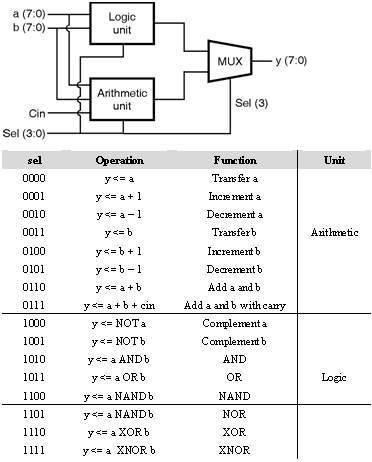Home > VHDL > Arithmetic Circuits > ALU

# Arithmetic Logic Unit

An Arithmetic Logic Unit is shown in figure below. It is a circuit of executing both arithmetic and logical operations. The operation is explained in the truth table. The output is selected by the MSB of sel, whereas the specific operation is selected by sel's other three bits. Two signals, arith and logic, are used to hold the results from the arithmetic and logic units.```LIBRARY ieee;
USE ieee.std_logic_1164.all;
USE ieee.std_logic_unsigned.all;
ENTITY ALU IS
PORT (a, b: IN STD_LOGIC_VECTOR (7 DOWNTO 0);
sel: IN STD_LOGIC_VECTOR (3 DOWNTO 0);
cin: IN STD_LOGIC;
y: OUT STD_LOGIC_VECTOR (7 DOWNTO 0));
END ALU;
ARCHITECTURE dataflow OF ALU IS
SIGNAL arith, logic: STD_LOGIC_VECTOR (7 DOWNTO 0);
BEGIN
WITH sel(2 DOWNTO 0) SELECT
arith <= a WHEN "000",
a+'1' WHEN "001",
a-'1' WHEN "010",
b WHEN "011",
b+'1' WHEN "100",
b-'1' WHEN "101",
a+b WHEN "110",
a+b+cin WHEN OTHERS;
WITH sel(2 DOWNTO 0) SELECT
logic <= NOT a WHEN "000",
NOT b WHEN "001",
a AND b WHEN "010",
a OR b WHEN "011",
a NAND b WHEN "100",
a NOR b WHEN "101",
a XOR b WHEN "110",
NOT (a XOR b) WHEN OTHERS;
WITH sel(3) SELECT
y <= arith WHEN '0',
logic WHEN OTHERS;
END dataflow;
```

## ALU using structural modeling

The ALU (Arithmetic Logic Unit) was presented in above figure. In that example, it assumed that the library contains the three components that are logic_unit, arith_unit, and mux. Here, alu.vhd,code is designed with the three components mentioned above. As can be seen, the COMPONENTS are declared in the main code itself. arith_unit

```arith_unit
LIBRARY ieee;
USE ieee.std_logic_1164.all;
USE ieee.std_logic_unsigned.all;
ENTITY arith_unit IS
PORT ( a, b: IN STD_LOGIC_VECTOR (7 DOWNTO 0);
sel: IN STD_LOGIC_VECTOR (2 DOWNTO 0);
cin: IN STD_LOGIC;
x: OUT STD_LOGIC_VECTOR (7 DOWNTO 0));
END arith_unit;
ARCHITECTURE arith_unit OF arith_unit IS
SIGNAL arith, logic: STD_LOGIC_VECTOR (7 DOWNTO 0);
BEGIN
WITH sel SELECT
x <= a WHEN "000",
a+1 WHEN "001",
a-1 WHEN "010",
b WHEN "011",
b+1 WHEN "100",
b-1 WHEN "101",
a+b WHEN "110",
a+b+cin WHEN OTHERS;
END arith_unit;
logic_unit
LIBRARY ieee;
USE ieee.std_logic_1164.all;
ENTITY logic_unit IS
PORT ( a, b: IN STD_LOGIC_VECTOR (7 DOWNTO 0);
sel: IN STD_LOGIC_VECTOR (2 DOWNTO 0);
x: OUT STD_LOGIC_VECTOR (7 DOWNTO 0));
END logic_unit;
ARCHITECTURE logic_unit OF logic_unit IS
BEGIN
WITH sel SELECT
x <= 	NOT a WHEN "000",
NOT b WHEN "001",
a AND b WHEN "010",
a OR b WHEN "011",
a NAND b WHEN "100",
a NOR b WHEN "101",
a XOR b WHEN "110",
NOT (a XOR b) WHEN OTHERS;
END logic_unit;
mux
LIBRARY ieee;
USE ieee.std_logic_1164.all;
ENTITY mux IS
PORT ( a, b: IN STD_LOGIC_VECTOR (7 DOWNTO 0);
sel: IN STD_LOGIC;
x: OUT STD_LOGIC_VECTOR (7 DOWNTO 0));
END mux;
ARCHITECTURE mux OF mux IS
BEGIN
WITH sel SELECT
x <= 	a WHEN '0',
b WHEN OTHERS;
END mux;
ALU code
LIBRARY ieee;
USE ieee.std_logic_1164.all;
ENTITY alu IS
PORT ( a, b: IN STD_LOGIC_VECTOR(7 DOWNTO 0);
cin: IN STD_LOGIC;
sel: IN STD_LOGIC_VECTOR(3 DOWNTO 0);
y: OUT STD_LOGIC_VECTOR(7 DOWNTO 0));
END alu;
ARCHITECTURE alu OF alu IS
COMPONENT arith_unit IS
PORT ( a, b: IN STD_LOGIC_VECTOR(7 DOWNTO 0);
cin: IN STD_LOGIC;
sel: IN STD_LOGIC_VECTOR(2 DOWNTO 0);
x: OUT STD_LOGIC_VECTOR(7 DOWNTO 0));
END COMPONENT;
COMPONENT logic_unit IS
PORT ( a, b: IN STD_LOGIC_VECTOR(7 DOWNTO 0);
sel: IN STD_LOGIC_VECTOR(2 DOWNTO 0);
x: OUT STD_LOGIC_VECTOR(7 DOWNTO 0));
END COMPONENT;
COMPONENT mux IS
PORT ( a, b: IN STD_LOGIC_VECTOR(7 DOWNTO 0);
sel: IN STD_LOGIC;
x: OUT STD_LOGIC_VECTOR(7 DOWNTO 0));
END COMPONENT;
SIGNAL x1, x2: STD_LOGIC_VECTOR(7 DOWNTO 0);
BEGIN
U1: arith_unit PORT MAP (a, b, cin, sel(2 DOWNTO 0), x1);
U2: logic_unit PORT MAP (a, b, sel(2 DOWNTO 0), x2);
U3: mux PORT MAP (x1, x2, sel(3), y);
END alu;
```